## Control Chart Execution by Using Temporal Logic

Temporal logic controls the execution of a chart in terms of time. In state actions and transitions, you can use two types of temporal logic:

• Event-based temporal logic tracks recurring events. You can use any explicit or implicit event as a base event.

• Absolute-time temporal logic tracks the elapsed time since a state became active. The timing for absolute-time temporal logic operators depends on the type of Stateflow® chart:

• Charts in a Simulink® model define absolute-time temporal logic in terms of simulation time.

• Standalone charts in MATLAB® define absolute-time temporal logic in terms of wall-clock time, which is limited to 1 millisecond precision.

### Temporal Logic Operators

To define the behavior of a Stateflow chart based on temporal logic, use the operators listed in this table. These operators can appear in:

• State `on` actions

• Actions on transition paths that originate from a state

Each temporal logic operator has an associated state, which is the state in which the action appears or from which the transition path originates. The Stateflow chart resets the counter used by each operator every time that the associated state reactivates.

OperatorSyntaxDescriptionExample
`after`

`after(n,E)`

`n` is a positive integer or an expression that evaluates to a positive integer value.

`E` is the base event for the operator.

Returns `true` if the event `E` has occurred at least `n` times since the associated state became active. Otherwise, the operator returns `false`.

Display a status message when the chart processes a broadcast of the event `E`, starting on the third broadcast of `E` after the state became active.

``````on after(3,E): disp("ON");``````

Transition out of the associated state when the chart processes a broadcast of the event `E`, starting on the fifth broadcast of `E` after the state became active.

`after(5,E)`

`after(n,tick)`

`n` is a positive integer or an expression that evaluates to a positive integer value.

Returns `true` if the chart has woken up at least `n` times since the associated state became active. Otherwise, the operator returns `false`.

The implicit event `tick` is not supported when a Stateflow chart in a Simulink model has input events. For more information, see Control Chart Behavior by Using Implicit Events.

Transition out of the associated state when the chart wakes up for at least the seventh time since the state became active, but only if the variable `temp` is greater than 98.6.

`after(7,tick)[temp > 98.6]`

`after(n,sec)`

`after(n,msec)`

`after(n,usec)`

`n` is a positive real number or an expression that evaluates to a positive real value.

Returns `true` if at least `n` units of time have elapsed since the associated state became active. Otherwise, the operator returns `false`.

In charts in a Simulink model, specify time in seconds (`sec`), milliseconds (`msec`), or microseconds (`usec`).

In standalone charts in MATLAB, specify time in seconds (`sec`). The operator creates a MATLAB `timer` object that generates an implicit event to wake up the chart. MATLAB `timer` objects are limited to 1 millisecond precision. For more information, see Events in Standalone Charts.

Set the `temp` variable to `LOW` every time that the chart wakes up, starting when the associated state is active for at least 12.3 seconds.

``````on after(12.3,sec): temp = LOW;``````
`at`

`at(n,E)`

`n` is a positive integer or an expression that evaluates to a positive integer value.

`E` is the base event for the operator.

Returns `true` if the event `E` has occurred exactly `n` times since the associated state became active. Otherwise, the operator returns `false`.

Display a status message when the chart processes the third broadcast of the event `E` after the state became active.

``````on at(3,E): disp("ON");``````

Transition out of the associated state when the chart processes the fifth broadcast of the event `E` after the state became active.

`at(5,E)`

`at(n,tick)`

`n` is a positive integer or an expression that evaluates to a positive integer value.

Returns `true` if the chart has woken up exactly `n` times since the associated state became active. Otherwise, the operator returns `false`.

The implicit event `tick` is not supported when a Stateflow chart in a Simulink model has input events. For more information, see Control Chart Behavior by Using Implicit Events.

Transition out of the associated state when the chart wakes up for the seventh time since the state became active, but only if the variable `temp` is greater than 98.6.

`at(7,tick)[temp > 98.6]`

`at(n,sec)`

`n` is a positive real number or an expression that evaluates to a positive real value.

Returns `true` if exactly `n` seconds have elapsed since the associated state became active. Otherwise, the operator returns `false`.

Using `at` as an absolute-time temporal logic operator is supported only in standalone charts in MATLAB. The operator creates a MATLAB `timer` object that generates an implicit event to wake up the chart. MATLAB `timer` objects are limited to 1 millisecond precision. For more information, see Events in Standalone Charts.

Set the `temp` variable to `HIGH` if the state has been active for exactly 12.3 seconds.

``````on at(12.3,sec): temp = HIGH;``````
`before`

`before(n,E)`

`n` is a positive integer or an expression that evaluates to a positive integer value.

`E` is the base event for the operator.

Returns `true` if the event `E` has occurred fewer than `n` times since the associated state became active. Otherwise, the operator returns `false`.

The temporal logic operator `before` is supported only in Stateflow charts in Simulink models.

Display a status message when the chart processes the first and second broadcasts of the event `E` after the state became active.

``````on before(3,E): disp("ON");``````

Transition out of the associated state when the chart processes a broadcast of the event `E`, but only if the state has been active for fewer than five broadcasts of `E`.

`before(5,E)`

`before(n,tick)`

`n` is a positive integer or an expression that evaluates to a positive integer value.

Returns `true` if the chart has woken up fewer than `n` times since the associated state became active. Otherwise, the operator returns `false`.

The implicit event `tick` is not supported when a Stateflow chart in a Simulink model has input events. For more information, see Control Chart Behavior by Using Implicit Events.

The temporal logic operator `before` is supported only in Stateflow charts in Simulink models.

Transition out of the associated state when the chart wakes up, but only if the variable `temp` is greater than 98.6 and the chart has woken up fewer than seven times since the state became active.

`before(7,tick)[temp > 98.6]`

`before(n,sec)`

`before(n,msec)`

`before(n,usec)`

`n` is a positive real number or an expression that evaluates to a positive real value.

Returns `true` if fewer than `n` units of time have elapsed since the associated state became active. Otherwise, the operator returns `false`.

Specify time in seconds (`sec`), milliseconds (`msec`), or microseconds (`usec`).

The temporal logic operator `before` is supported only in Stateflow charts in Simulink models.

Set the `temp` variable to `MED` every time that the chart wakes up, but only if the associated state has been active for fewer 12.3 seconds.

``````on before(12.3,sec): temp = MED;``````
`every`

`every(n,E)`

`n` is a positive integer or an expression that evaluates to a positive integer value.

`E` is the base event for the operator.

Returns `true` at every `n`th occurrence of the event `E` since the associated state became active. Otherwise, the operator returns `false`.

Display a status message when the chart processes every third broadcast of the event `E` after the state became active.

``````on every(3,E): disp("ON");``````

Transition out of the associated state when the chart processes every fifth broadcast of the event `E` after the state became active.

`every(5,E)`

`every(n,tick)`

`n` is a positive integer or an expression that evaluates to a positive integer value.

Returns `true` at every `n`th time that the chart wakes up since the associated state became active. Otherwise, the operator returns `false`.

The implicit event `tick` is not supported when a Stateflow chart in a Simulink model has input events. For more information, see Control Chart Behavior by Using Implicit Events.

Transition out of the associated state every seventh `tick` event since the state became active, but only if the variable `temp` is greater than 98.6.

`every(7,tick)[temp > 98.6]`

`every(n,sec)`

`n` is a positive real number or an expression that evaluates to a positive real value.

Returns `true` every `n` seconds since the associated state became active. Otherwise, the operator returns `false`.

Using `every` as an absolute-time temporal logic operator is supported only in standalone charts in MATLAB. The operator creates a MATLAB `timer` object that generates an implicit event to wake up the chart. MATLAB `timer` objects are limited to 1 millisecond precision. For more information, see Events in Standalone Charts.

Increment the `temp` variable by 5 every 12.3 seconds that the state is active.

``````on every(12.3,sec): temp = temp+5;``````
`temporalCount`

`temporalCount(E)`

`E` is the base event for the operator.

Returns the number of occurrences of the event `E` since the associated state became active.

Using `temporalCount` as an event-based temporal logic operator is supported only in Stateflow charts in Simulink models.

Access successive elements of the array `M` each time that the chart processes a broadcast of the event `E`.

``````on E: y = M(temporalCount(E));``````
`temporalCount(tick)`

Returns the number of times that the chart has woken up since the associated state became active.

The implicit event `tick` is not supported when a Stateflow chart in a Simulink model has input events.

Using `temporalCount` as an event-based temporal logic operator is supported only in Stateflow charts in Simulink models.

Store the value of the input data `u` in successive elements of the array `M`.

```en,du: M(temporalCount(tick)+1) = u;```

`temporalCount(sec)`

`temporalCount(msec)`

`temporalCount(usec)`

Returns the length of time that has elapsed since the associated state became active.

Specify time in seconds (`sec`), milliseconds (`msec`), or microseconds (`usec`).

Store the number of milliseconds since the state became active.

```en,du: y = temporalCount(msec);```
`elapsed`

`elapsed(sec)`

Returns the length of time that has elapsed since the associated state became active.

Equivalent to `temporalCount(sec)`.

Store the number of seconds since the state became active.

```en,du: y = elapsed(sec);```
`et`An alternative way to execute `elapsed(sec)`.

When the chart processes a broadcast of the event `E`, transition out of the associated state and display the elapsed time since the state became active.

`E{disp(et);}`
`count`

`count(C)`

`C` is an expression that evaluates to `true` or `false`.

Returns the number of times that the chart has woken up since the conditional expression `C` became `true` and the associated state became active.

The Stateflow chart resets the value of the `count` operator if the conditional expression `C` becomes `false` or if the associated state becomes inactive.

In charts in a Simulink model, the value of `count` may depend on the step size. Changing the solver or step size for the model affects the results produced by the `count` operator.

Transition out of the associated state when the variable `x` has been greater than or equal to 2 for longer than five chart executions.

`[count(x>=2) > 5]`

Store the number of chart executions since the variable `x` became greater than 5.

```en,du: y = count(x>5);```
`duration`

`duration(C)`

`duration(C,sec)`

`duration(C,msec)`

`duration(C,usec)`

• `C` is an expression that evaluates to `true` or `false`.

Returns the length of time that has elapsed since the conditional expression `C` became `true` and the associated state became active.

Specify time in seconds (`sec`), milliseconds (`msec`), or microseconds (`usec`). The default unit is seconds.

The Stateflow chart resets the value of the `duration` operator if the conditional expression `C` becomes `false` or if the associated state becomes inactive.

The temporal logic operator `duration` is not supported in standalone charts in MATLAB.

Transition out of the state when the variable `x` has been greater than or equal to 0 for longer than 0.1 seconds.

`[duration(x>=0) > 0.1]`

Store the number of milliseconds since the variable `x` became greater than 5 and the state became active.

```en,du: y = duration(x>5,msec);```

You can use quotation marks to enclose the keywords `'tick'`, `'sec'`, `'msec'`, and `'usec'`. For example, `after(5,'tick')` is equivalent to `after(5,tick)`.

Note

The temporal logic operators `after`, `at`, `before`, and `every` compare the threshold `n` to an internal counter of integer type. If `n` is a fixed-point number defined by either a slope that is not an integer power of two or a nonzero bias, then the comparison can yield unexpected results due to rounding. For more information, see Relational Operations for Fixed-Point Data.

### Examples of Temporal Logic

#### Define Time Delays

This example shows how to define two absolute time delays in a continuous-time chart.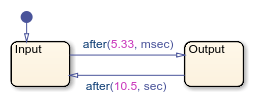The execution of the chart follows these steps:

1. When the chart awakens, the state `Input` activates first.

2. After 5.33 milliseconds of simulation time, the transition from `Input` to `Output` occurs.

3. The state `Input` becomes inactive and the state `Output` becomes active.

4. After 10.5 seconds of simulation time, the transition from `Output` to `Input` occurs.

5. The state `Output` becomes inactive and the state `Input` becomes active.

Steps 2 through 5 are repeated until the simulation ends.

If a chart has a discrete sample time, any action in the chart occurs at integer multiples of this sample time. For example, if the Simulink® solver uses a fixed step of size 0.1 seconds, the first transition from state `Input` to state `Output` occurs at t = 0.1 seconds. This behavior applies because the solver does not wake the chart at exactly t = 5.33 milliseconds. Instead, the solver wakes the chart at integer multiples of 0.1 seconds, such as t = 0.0 and 0.1 seconds.

#### Detect Elapsed Time

In this example, a Step (Simulink) block provides a unit step input to a Stateflow chart.The chart determines when the input `u` equals 1:

• If the input equals 1 before t = 2 seconds, a transition occurs from `Start` to `Fast`.

• If the input equals 1 between t = 2 and t = 5 seconds, a transition occurs from `Start` to `Medium`.

• If the input equals 1 after t = 5 seconds, a transition occurs from `Start` to `Slow`.

#### Use Absolute-Time Temporal Logic in an Enabled Subsystem

You can use absolute-time temporal logic in a chart that resides in a conditionally executed subsystem. When the subsystem is disabled, the chart becomes inactive and the temporal logic operator pauses while the chart is asleep. The operator does not continue to count simulation time until the subsystem is reenabled and the chart is awake.

This model has an enabled subsystem with the States when enabling parameter set to `held`.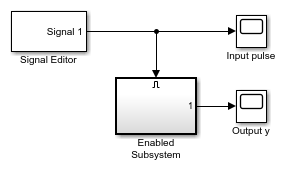The subsystem contains a chart that uses the `after` operator to trigger a transition.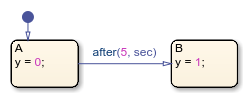The Signal Editor (Simulink) block provides an input signal with these characteristics:

• The signal enables the subsystem at t = 0.

• The signal disables the subsystem at t = 2.

• The signal reenables the subsystem at t = 6.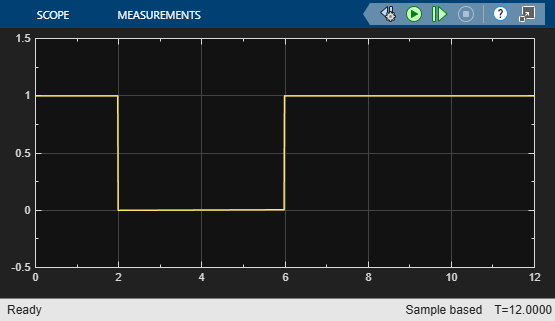This graph shows the total time elapsed in the chart. When the input signal enables the subsystem at time t = 0, state `A` becomes active. While the system is enabled, the elapsed time increases. When the subsystem is disabled at t = 2, the chart goes to sleep and the elapsed time stops increasing. For 2 < t < 6, the elapsed time stays frozen at 2 seconds because the system is disabled. When the chart wakes up at t = 6, elapsed time starts to increase again.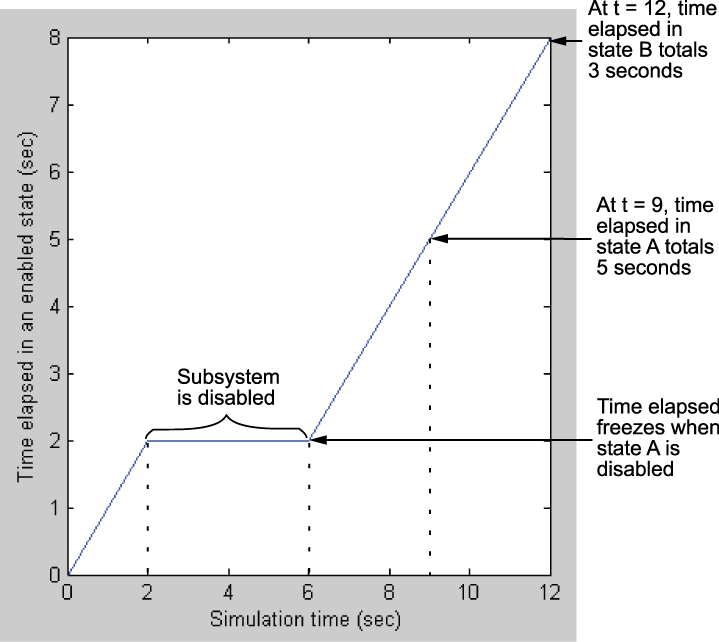The transition from state `A` to state `B` depends on the elapsed time while state `A` is active, not on the simulation time. Therefore, the transition occurs at t = 9, when the elapsed time in state `A` equals 5 seconds. When the transition occurs, the output value `y` changes from 0 to 1.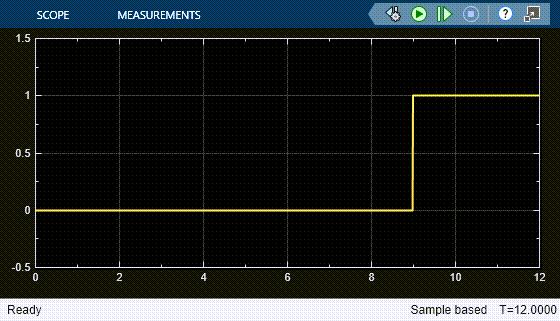This model behavior applies only to subsystems where you set the Enable block parameter States when enabling to `held`. If you set the parameter to `reset`, the chart reinitializes completely when the subsystem is reenabled. Default transitions execute and any temporal logic counters reset to 0.

### Notation for Event-Based Temporal Logic in Transitions

In Stateflow charts in Simulink models, the operators `after`, `at`, and `before` support two distinct notations to express event-based temporal logic in a transition.

• Trigger notation defines a transition that depends only on the base event for the temporal logic operator. Trigger notation follows this syntax:

`temporalLogicOperator(n,E)[C]`
where:

• `temporalLogicOperator` is a Boolean temporal logic operator.

• `n` is the occurrence count of the operator.

• `E` is the base event of the operator.

• `C` is an optional condition expression.

When you use trigger notation, the transition can occur only when the chart processes a broadcast of the base event `E`.

• Conditional notation defines a transition that depends on base and nonbase events. Conditional notation follows this syntax:

`F[temporalLogicOperator(n,E) && C]`
where:

• `temporalLogicOperator` is a Boolean temporal logic operator.

• `n` is the occurrence count of the operator.

• `E` is the base event of the operator.

• `F` is an optional nonbase event.

• `C` is an optional condition expression.

When you use conditional notation with a nonbase event `F`, the transition can occur only when the chart processes a broadcast of `F`. If you omit the nonbase event, the transition can occur when the chart is processing any explicit or implicit event.

Conditional notation for temporal logic operators is not supported in standalone charts in MATLAB.

For example, this transition label uses trigger notation to indicate a transition out of the associated state when the chart processes a broadcast of the base event `E`, starting on the fifth broadcast of `E` after the state became active.

`after(5,E)`

In contrast, this transition label uses conditional notation to indicate a transition out of the associated state when the state has been active for at least five broadcasts of the base event `E`, even if the chart is not processing a broadcast of `E`.

`[after(5,E)]`

Note

The operator `every` supports trigger and conditional notations. However, both notations are equivalent for this operator. The transition labels `every(5,E)` and `[every(5,E)]` indicate a transition out of the associated state when the chart processes the kth broadcast of the base event `E` after the state became active, where k is a multiple of five.

### Best Practices for Temporal Logic

#### Do Not Use Temporal Logic on Transition Paths Without A Source State

The value of a temporal logic operator depends on when its associated state became active. To ensure that every temporal logic operator has a unique associated state, only use these operators in:

• State `on` actions

• Actions on transition paths that originate from a state

Do not use temporal logic operators on default transitions or on transitions in graphical functions because these transitions do not originate from a state.

#### Use Absolute-time Temporal Logic Instead of `tick` in Charts in Simulink Models

In charts in a Simulink model, the value of delay expressions that use absolute-time temporal logic are semantically independent of the sample time of the model. In contrast, delay expressions that use temporal logic based on the implicit event `tick` depend on the step size used by the Simulink solver.

Additionally, absolute-time temporal logic is supported in charts that have input events. The implicit event `tick` is not supported when a Stateflow chart in a Simulink model has input events.

#### Do Not Use `at` for Absolute-Time Temporal Logic in Charts in Simulink Models

In charts in a Simulink model, using `at` as an absolute-time temporal logic operator is not supported. Instead, use the `after` operator. For example, suppose that you want to define a time delay using the expression `at(5.33,sec)`.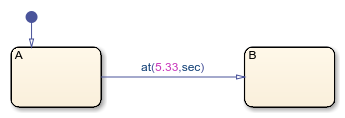To prevent a run-time error, change the transition label to `after(5.33,sec)`.#### Unexpected Results for Large Parameter Values

A Stateflow absolute time temporal logic condition such as `after(x,sec)` might not evaluate to `true` at the expected time after entering a state with the following conditions:

• The chart has a periodic discrete sample time.

• The chart logic makes the state remain active for greater than `2147418` units of time. The units of time are the smallest time units in any temporal logic expression used by that state. For example, if the state has two outgoing transitions, one using `after(x,sec)` and the other using `after(x,msec)`, the units of time are ```msec (milliseconds)```.

Typically, unexpected results occur when the length of time in the state is bigger than `2147418` units of time. However, this may change depending on the sample time of the chart.

#### Do Not Use `every` for Absolute-Time Temporal Logic in Charts in Simulink Models

In charts in a Simulink model, using `every` as an absolute-time temporal logic operator is not supported. Instead, use an outer self-loop transition with the `after` operator. For example, suppose that you want to print a status message for an active state every 2.5 seconds during chart execution.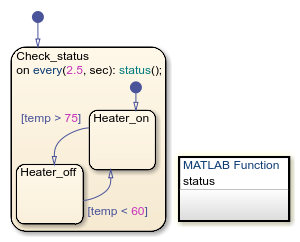To prevent a run-time error, replace the state action with an outer self-loop transition.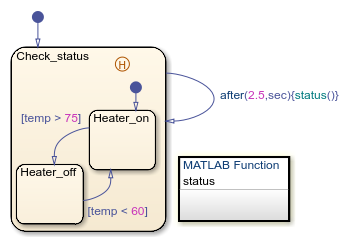Add a history junction in the state so the chart remembers the state settings prior to each self-loop transition. See Resume Prior Substate Activity by Using History Junctions.

#### Do Not Use Temporal Logic in Transition Paths with Multiple Sources in Standalone Charts in MATLAB

Standalone charts in MATLAB do not support the use of temporal logic operators on transition paths that have more than one source state. For example, this standalone chart produces a run-time error because the temporal logic expression `after(10,sec)` triggers a transition path that has more than one source state.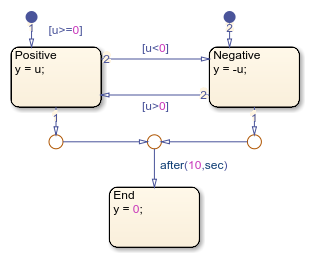To resolve the issue, use temporal logic expressions on separate transition paths, each with a single source state.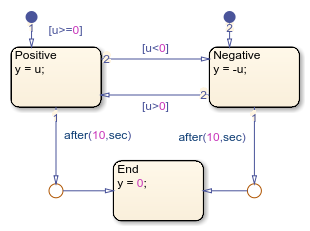#### Avoid Mixing Absolute-time Temporal Logic and Conditions in Transition Paths of Standalone Charts in MATLAB

In standalone charts in MATLAB, the operators `after`, `at`, and `every` create MATLAB `timer` objects that generate implicit events to wake up the chart. Combining these operators with conditions on the same transition path can result in unintended behavior:

• If a condition on the transition path is false when the `timer` wakes up the chart, the chart performs the `during` and `on` actions of the active state.

• The chart does not reset the `timer` object associated with the operators `after` and `at`. If the condition on the transition path becomes true at a later time, the transition does not take place until another explicit or implicit event wakes up the chart.

For example, in this chart, the transition path from state `A` to state `B` combines the absolute-time temporal logic trigger `after(1,sec)` and the condition `[guard]`. The transition from state `A` to state `C` has the absolute-time temporal logic trigger `after(5,sec)`. Each transition is associated with a `timer` object that generates an implicit event. Initially, the local variable `guard` is `false`.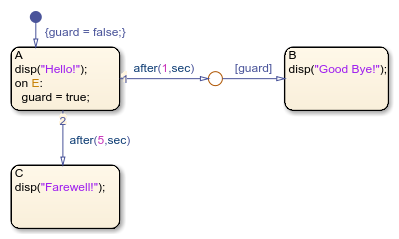When you execute the chart, state `A` becomes active. The chart performs the `entry` action and displays the message `Hello!`. After 1 second, the `timer` associated with the transition from `A` to `B` wakes up the chart. Because the transition is not valid, the chart executes the `during` action in state `A` and displays the message `Hello!` a second time.

Suppose that, after 2 seconds, the chart receives the input event `E`. The chart executes the `on` action in state `A` and changes the value of `guard` to `true`. Because the chart does not reset the `timer` associated with the operator `after`, the transition from `A` to `B` does not take place until another event wakes up the chart.

After 5 seconds, the `timer` associated with the transition from `A` to `C` wakes up the chart. Because the transition from `A` to `B` is valid and has a higher execution order, the chart does not take the transition to state `C` or display the message `Farewell!`. Instead, state `B` becomes active and the chart displays the message `Good bye!`.

#### Use Charts with Discrete Sample Times for More Efficient Code Generation

The code generated for discrete charts that are not inside a triggered or enabled subsystem uses integer counters to track time instead of the time provided by Simulink. This behavior allows for more efficient code generation in terms of overhead and memory, and enables this code for use in software-in-the-loop (SIL) and processor-in-the-loop (PIL) simulation modes. For more information, see SIL and PIL Simulations (Embedded Coder).